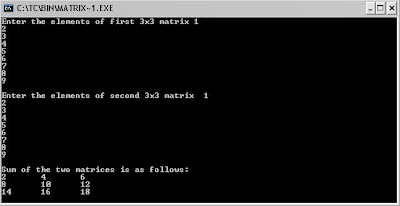## Saturday, December 4, 2010

### C program to find the sum of two matrix

Program Code
`//C program to find the sum of two matrix#include < stdio.h >#include< conio.h >void main(){int a, b, c, i, j;clrscr();printf("Enter the elements of first 3x3 matrix");for (i = 0;i < 3; i++){  for (j = 0; j < 3; j++)  {   scanf("%d",&a[i][j]);  }}printf("\nEnter the elements of second 3x3 matrix");for (i = 0; i < 3; i++){  for (j = 0; j < 3; j++)  {   scanf("%d", &b[i][j]);  }}printf("\nSum of the two matrices is as follows:");for (i = 0;i < 3; i++){ printf("\n"); for (j = 0; j < 3; j++)  {   c[i][j] = a[i][j] + b[i][j];   printf("%d \t", c[i][j]);  }}getch();}`

Input :
Enter the elements of first 3x3 matrix
1
2
3
4
5
6
7
8
9
Enter the elements of second 3x3 matrix
1
2
3
2
3
1
3
1
2

Ouput :
Sum of the two matrices is as follows
2 4 6
6 8 7
10 9 11